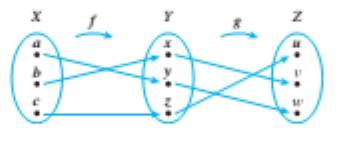Chapter 7.3, Problem 26ES### Discrete Mathematics With Applicat...

5th Edition
EPP + 1 other
ISBN: 9781337694193

#### Solutions

Chapter
Section### Discrete Mathematics With Applicat...

5th Edition
EPP + 1 other
ISBN: 9781337694193
Textbook Problem
4 views

# In 26 and 27 find ( g ∘ f ) − 1 , g − 1 , f − 1 , and f − 1 ∘ g − 1 , and state how ( g ∘ f ) − 1 and f − 1 ∘ g − 1 are related.Let X = { a , b , c } ,     Y = { x , y , z } , and Z = { u , v , w } . Define f : X → Y and g : Y → Z by the arrow diagrams below.To determine

To find gof,(gof)1,g1,f1and f1og1, and explain how (g of)1 and f1o g1 are related.

Explanation

Given information:

Let X={a,c,b}, Y={x,y,z} and Z={u,v,w}. Define f:XY and g:YZ by the arrow diagrams below.

Concept used:

A function is said to be one to one function if the element in domain must be mapped with distinct

Element in codomain.

A function is onto function if element in codomain is mapped with element in domain.

Calculation:

f(a)=y,g(y)=w          gf(a)=g{f(a)}=g{y}=wf(b)=g,g(x)=v            gf(b)=g{f(b)}=g(x)=vf(c)=z,g(z)=u              gf(c)=g{f(c)}=g{z}=u

gf={(a,w),(b,v),(c,u)}(gf)1={(w

### Still sussing out bartleby?

Check out a sample textbook solution.

See a sample solution

#### The Solution to Your Study Problems

Bartleby provides explanations to thousands of textbook problems written by our experts, many with advanced degrees!

Get Started